## Oscillators and Monostable using the 4093 IC – Part 2 (ART538E)

This is the second of two articles were we describe basic configurations of oscillators, monostables and biestables circuits using the 4093 IC. The article is part of my book CMOS Projects and Experiments using the 4093 (out of print).

## Two-Gate Oscillator

Two NAND Schmitt triggers of a 4093 can create a two-gate oscillator, as shown in Fig. 1. This circuit generates a square wave with excellent thermal stability and operates in a large range of frequencies. With the values shown in Fig. 15, it operates at about 1 kHz.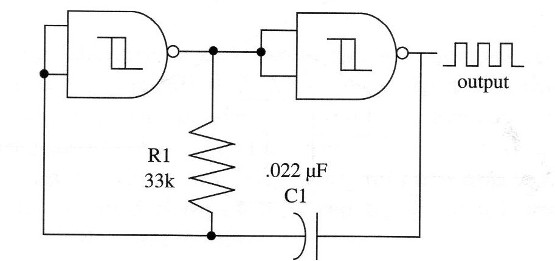Figure 1 – Two- gate oscillator runing at 1 kHz

In practice, C1 must be a nonpolarized capacitor and can have any value from 50 pF to some microfarads. R1 can have any value in the range between 4.7 k and 20 M. As in other applications, for a variable frequency operation, Wire a fixed and a variable resistor in series in the R1 position.

The voltage in the junction of C1 and R1 is prevented from swing below zero or above the positive supply level by the built-in clamping diodes at the 4093 inputs, which causes the operating frequency of the circuit to be somewhat dependent on the power supply voltage. A simple rule shows that the frequency falls about 0.1 percent for each 1 percent rise in supply voltage.

The deficiencies of the basic circuit are minimized by using a compensated configuration as shown in Fig. 2. In this circuit, R2 is wired in series with the first gate input, and it allows the R1-C1 junction to swing freely below zero and above the positive supply. Values for this component typically range between 2 and 10 times R1.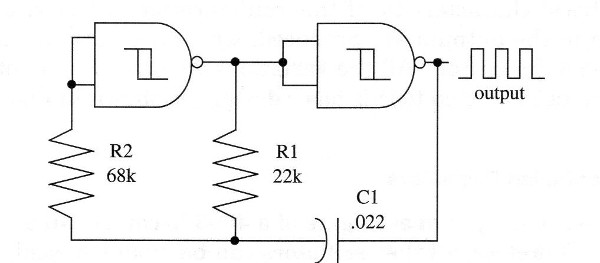Figure 2 – Improved two-gate oscillator

In this circuit, frequency varies only 0.5 percent when the supply voltage rises from 5 to 15 V. Other components can be added to the basic circuit for better performance, as shown in Fig. 3.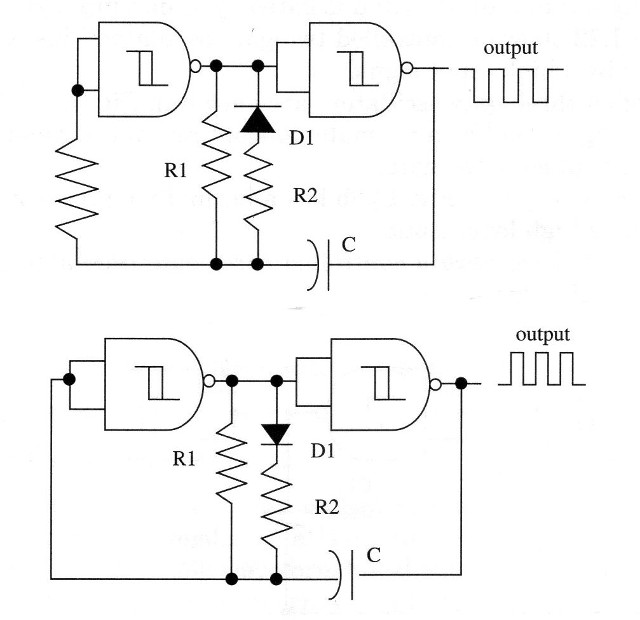Figure 3 – Diodes can be added to a non-symetrical output

Diodes can be used to give a nonsymmetrical output, as we will see in some practical applications.

As we have a signal traveling through two gates rather than just one, this circuit is slower than the one-gate oscillator. The maximum practical operation frequency for a 6 V power supply is about 500 kHz.

One input of the gate can be used for control em shields. (see previous configurations)

## Three-Gate Oscillator

Three of the four gates of a 4093 can be used to make an oscillator with very clean output waveform, as shown in Fig. 4.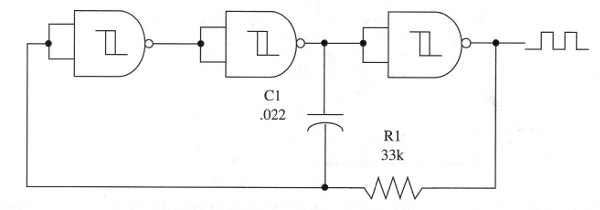Figure 4 – A three-gate oscillator using the 4093 IC

Two gates as inverters wired in series give a high-gain noninverting stage and, due the electrical characteristic of this configuration, a fast rise time and fall time in the outputs are produced, which are directly suitable for use as clock generators. All the variations we have seen in other oscillators can be subjected to this basic design, as shown in Fig. 5.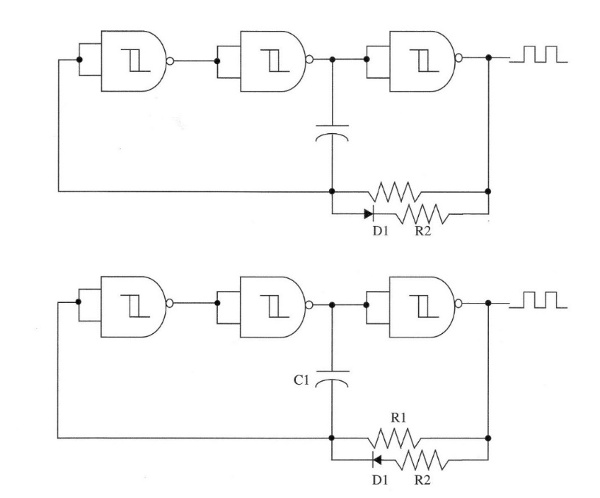Figure 5 – Asymetric outputs produced by diodes

## Controlled Oscillators

We can use one input of each gate of a 4093 to control all oscillator operations. Therefore, all the oscillators can be modified easily for gated operation, so they can be turned on and off via an external signal.

A microcontroller can be used to this task allowing the use of the 4093 in shields without the need of aditional circuits. In this case, it must be observed that the power supply voltage for the 4093 must be the same ot he microcontroller or the used logic output (3,3 or 5 V).

In Fig.6 we show a one-gate controlled oscillator. This circuit has a normally low output (0 V) and is gated by a high input signal.Figure 6 – Gated or triggered one-gate oscillator using the 4093.

Figure 7 shows a controlled two-gate oscillator. This oscillator is turned on by a high input signal.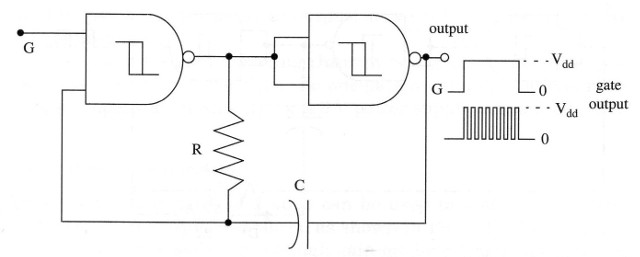Figure 7 – Gated two-gate oscillator using the 4093 IC

Controlled three-gate oscillators are shown in Fig. 8. The circuit shown in Fig. 8a has a normally low output and is gated by a high logic level applied to the gate. The circuit shown in Fig. 8b has a normally high output and also

is gated by a high logic input.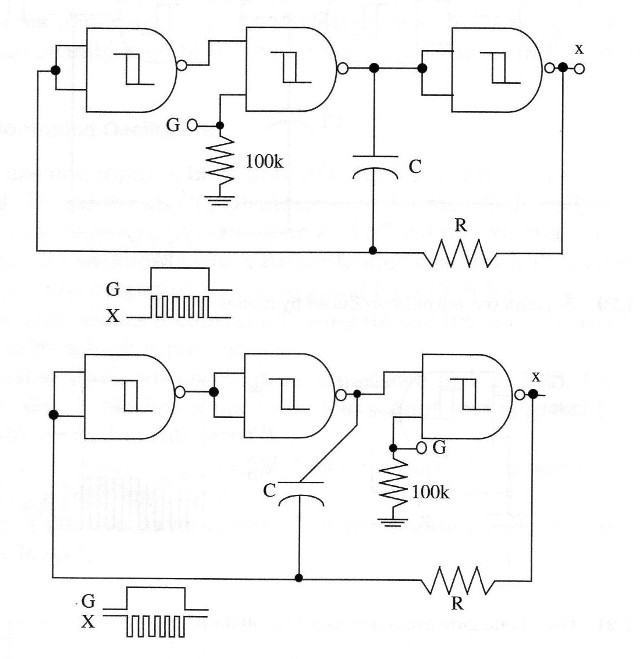Figure 8 – Three-gate controlled oscillator using the 4093 IC

This control can be made by a microcontroller such as the Arduino, PIC or other. Remember that the 4093 must be supplied by the same voltage used to power the microcontroller or available in the control output (3,3 or 5 V). A common ground for the microcontroller and the 4093 is necessary.